# Acids and Bases

## What Is An Acid Or A Base?

By the 1884 definition of Svante Arrhenius (Sweden), an acid
is a material that can release a proton or hydrogen ion (H
+
). Hydrogen chloride in water solution ionizes
and becomes hydrogen ions and chloride ions. If that is the case, a base,
or alkali, is a material that can donate a hydroxide ion (OH).
Sodium hydroxide in water solution becomes sodium ions and hydroxide ions. By
the definition of both Thomas Lowry (England) and J.N. Br¯nsted (Denmark)
working independently in 1923, an acid is a material that donates a proton
and a base is a material that can accept a proton. Was Arrhenius erroneous? \$| 😎
No. The Arrhenius definition serves well for a limited use. We are going to
use the Arrhenius definitions most of the time. The Lowry- Br¯nsted definition
is broader, including some ideas that might not initially seem to be acid and
base types of interaction. Every ion dissociation that involves a hydrogen or
hydroxide ion could be considered an acid- base reaction. Just as with the
Arrhenius definition, all the familiar materials we call acids are also acids
in the Lowry – Br¯nsted model. The G.N. Lewis (1923) idea of acids and bases
is broader than the Lowry – Br¯nsted model. The Lewis definitions are: Acids
are electron pair acceptors and bases are electron pair donors.

We can consider the same idea in the Lowry – Br¯nsted fashion.
Each ionizable pair has a proton donor and a proton acceptor. Acids are paired
with bases. One can accept a proton and the other can donate a proton. Each acid
has a proton available (an ionizable hydrogen) and another part, called
the conjugate base. (That word, ‘conjugate’ just
means that it "goes with" the other part.) When the acid ionizes, the hydrogen ion is
the acid and the rest of the original acid is the conjugate base. Nitric acid, HNO
3,
dissociates (splits) into a hydrogen ion and a nitrate ion. The
hydrogen almost immediately joins to a water molecule to make a hydronium ion. The nitrate
ion is the conjugate base of the hydrogen ion. In the second part of the reaction, water
is a base (because it can accept a proton) and the hydronium ion is its conjugate acid.

 HNO3 + H2O ===> (NO3)– + (H3O)+ ACID BASE CONJUGATE BASE CONJUGATE ACID

Chemists or chemistry texts often use the hydrogen ion, H+
to show a hydrogen ion released into water solution. In a way, there is no such thing as a hydrogen ion or proton without
anything else. The majority of hydrogen atoms are only a single proton and a single electron.
If you remove the electron to make it an ion, the only thing that is left is a proton.
Protons just don’t exist naked like that in water solution. Remember that
water is a very polar material. There is a strong
partial negative charge on the side of the oxygen atom and a strong partial positive
charge on the hydrogen side. Any loose hydrogen ion, having a positive charge,  would
quickly find itself near the oxygen of a water molecule. At close range from the
charge attraction, the hydrogen ion would find a pair (its choice of two pairs) of
unshared electrons around the oxygen that would be capable of filling the its outer shell.
Each hydrogen ion unites with a water molecule to produce a hydronium ion,
(H3O)+,
the real species that acts as acid. The hydroxide ion in solution does not combine
with a water molecule in any similar fashion. As we write reactions of acids and bases, it
is usually most convenient to ignore the hydronium ion in favor of writing just a hydrogen
ion, H+.

## Properties Of Acids

For the properties of acids and bases we will use the Arrhenius definitions.

Acids release a hydrogen ion into water (aqueous) solution. You will usually see the formula for an acid with the ionizable hydrogen at the beginning, such as HCl, hydrochloric acid, or H(C2H3O2), acetic acid.

Acids neutralize bases in a neutralization reaction. An acid and a base combine to make a
salt and water. A salt is any ionic compound that could be
made with the anion of an acid and the cation of a base. The hydrogen ion of the acid and
the hydroxide ion of the base unite to form water.

Acids corrode active metals. Even gold, the least active metal, is attacked by an acid,
a mixture of acids called ‘aqua regia,’ or ‘royal liquid.’ When an acid reacts
with a metal, it produces a compound with the cation of the metal and the anion of the acid and hydrogen
gas.

Acids turn blue litmus to red. Litmus is one of a large number of organic
compounds that change colors when a solution changes acidity at a particular
point. Litmus is the oldest known pH indicator. It is red in acid and blue in
base. The phrase, ‘litmus test,’ indicates that litmus has been around a long
time in the English language. Litmus does not change color exactly at the
neutral point between acid and base, but very close to it. Litmus is often
impregnated onto paper to make ‘litmus paper.’

Acids taste sour. TASTING LAB ACIDS IS NOT PERMITTED BY ANY SCHOOL. The word ‘sauer’ in
German means acid and is pronounced almost exactly the same way as ‘sour’ in English.
(Sauerkraut is sour cabbage, cabbage preserved in its own fermented lactic acid.
http://en.wikipedia.org/wiki/Sauerkraut
http://www.wildfermentation.com/resources.php?page=sauerkraut
Stomach acid is
hydrochloric acid. Although tasting stomach acid is not pleasant, it has the sour taste of
acid. Acetic acid is the acid ingredient in vinegar. Citrus fruits such as lemons,
grapefruit, oranges, and limes have citric acid in the juice. Sour milk, sour cream,
yogurt, kimchi, and cottage cheese have lactic acid from the fermentation of the sugar lactose.

## Properties Of Bases

Bases release a hydroxide ion into water solution. (Or, in the Lowry – Br¯nsted model,
cause a hydroxide ion to be released into water solution by accepting a hydrogen ion in water.)

Bases neutralize acids in a neutralization reaction. The word – reaction is:
Acid plus base makes water plus a salt.
Where ‘Y’ is the anion of acid ‘HY,’ and ‘X’ is the cation of base
‘XOH,’  and ‘XY’ is the salt in the product, the reaction is: HY + XOH ===> HOH + XY

Bases denature protein. This accounts for the "slippery" feeling on hands
when exposed to base. Strong bases

that dissolve in water well, such as sodium or
potassium lye are very dangerous because a great amount of the structural material of
human beings is made of protein. Serious damage to flesh can be avoided by careful use of
strong bases.

Bases turn red litmus to blue. This is not to say that litmus is the
only acid – base indicator, but that it is likely the oldest one.

Bases taste bitter. There are very few food materials that are alkaline, but those that
are taste bitter. It is even more important that care be taken in tasting bases. Again, NO
SCHOOL PERMITS TASTING OF LAB CHEMICALS. Tasting of bases is more dangerous than tasting
acids due to the property of stronger bases to denature protein.

## Strong Acids And Strong Bases

The common acids that are almost one hundred percent ionized are:
HNO3 – nitric acid
HCl1 – hydrochloric acid
H2SO4 – sulfuric acid
HClO4 – perchloric acid
HBr1 – hydrobromic acid
HI1 – hydroiodic acid

The acids on this short list are called strong acids, because
the amount of acid quality of a solution depends upon the concentration of ionized
hydrogens. Muriatic acid is the name given to an industrial grade of hydrochloric acid that is often
used in the finishing of concrete. Less concentated hydrochloric acid can be found in the human stomach.
Strong acids are completely ionized in water. You are not likely to see much HBr or HI in the lab because they are
expensive. You are not likely to see perchloric acid in a school setting because it can explode if not treated
carefully. Other acids are incompletely ionized, existing mostly as the unionized form.
Incompletely ionized acids are called weak acids, because there is a smaller
concentration of ionized hydrogens available in the solution. Do not confuse this
terminology with the concentration of acids. The differences in concentration of the
entire acid will be termed dilute or concentrated.

In the list of strong acids, sulfuric acid is the only one that is
diprotic, because it has two ionizable hydrogens per formula
(or two mols of ionizable hydrogen per mol of acid). (Sulfuric acid ionizes in two steps.
The first time a hydrogen ion splits off of the sulfuric acid, it acts like a strong acid.
The second time a hydrogen splits away from the sulfate ion, it acts like a weak acid.) The
other acids in the list are monoprotic, having only one ionizable
proton per formula. Phosphoric acid, H3PO4,
is a weak acid. Phosphoric acid has three hydrogen ions available to ionize and lose as a proton,
and so phosphoric acid is triprotic. We call any acid with two or more
ionizable hydrogens polyprotic.

Likewise, there is a short list of strong bases, ones that completely ionize into
hydroxide ions and a conjugate acid. All of the bases of Group I and Group II  metals
except for beryllium are strong bases. Again, like the strong acids, the strong bases are completely ionized in water solution. Lithium, rubidium and cesium hydroxides are not
often used in the lab because they are expensive. The bases of Group II metals, magnesium,
calcium, barium, and strontium are strong, but all of these bases have somewhat limited
solubility. Barium hydroxide has a high enough solubility to really call it the only dibasic
strong base. Magnesium hydroxide has a particularly small solubility. Potassium and
sodium hydroxides both have the common name of lye. Soda lye
(NaOH) and potash lye (KOH) are common names to distinguish the two compounds.

LiOH1 – lithium hydroxide
NaOH1 – sodium hydroxide
KOH1 – potassium hydroxide
RbOH1 – rubidium hydroxide
CsOH1 – cesium hydroxide
(Mg(OH)2 – magnesium hydroxide)
(Ca(OH)2 – calcium hydroxide)
(Sr(OH)2 – strontium hydroxide)
Ba(OH)2 – barium hydroxide

The bases of Group I metals are all monobasic. The bases of
Group II metals are all dibasic. Aluminum hydroxide,
Al(OH)3 is tribasic.
Any material with two or more ionizable hydroxyl groups would be called polybasic.
Most of the alkaline organic compounds (and some inorganic materials) have an amino group
-(NH2) rather than an ionizable hydroxyl
group. The amino group attracts a proton (hydrogen ion) to become -(NH3
)+.
(The dash before the (NH3)+
or (NH2) indicates a single
bonding electron, so this is attached to something else by a covalent bond.) By the Lowry- Br¯nsted
definition, an amino group definitely acts as a base, and the effect of removing hydrogen ions from
water molecules is the same as adding hydroxide ions to the solution.

Memorize the strong acids and strong bases. All other acids or bases are weak.

## Solubility And Dissociation

Now, after considering the bases of Group II metals, is a fine time to think about
acidity of a solution and the solubility of the compound. Calcium and magnesium hydroxides
are used in antacids, materials used to combat gastrointestinal
acidity. How can that be if they are strong bases? In order to act as a base, the material
must be dissolved. Almost all of these bases that are dissolved are dissociated, or
ionized, but the low solubility of these bases makes them safe to swallow. An acid or base
must first dissolve before it can dissociate (come apart) or ionize (become a pair of
ions).

It is important to notice that just because a compound has a hydrogen or an -OH group as
a part of the structure does not mean that it can be an acid or a base. The hydrogens of
methane, CH4, are all very covalently
attached to the carbon atom, and the hydrogens do not ionize, so methane is not an acid..
Glycerin (or glycerol) has three -OH groups in its structure, but the -OH groups do not
separate as an ion, so glycerin is not a base.

 CH2OH | CHOH | CH2OH

These are alcoholic -OH groups attached to a carbon atom. THE AVAILABILITY OF THE HYDROXIDE
OR HYDROGEN AS AN ION DEPENDS UPON WHAT IT IS ATTACHED TO.

The chemical equation for the dissociation of nitric acid is:For strong acids and strong bases the equation goes completely to the right. There
is none of the original acid or base, but only the ions of the material unattached to each
other in the water.

## Overview Of pH

pH is just an easy way to express how acidic or alkaline a water
solution is. The pH of a solution is the negative log of the hydrogen ion concentration.
The hydrogen ion concentration is inversely proportional to the hydroxide ion
concentration, and the two of them multiplied together give the number 1 E-14. It is a
little bit odd that the product (result of multiplication) of the molar concentration of
the hydrogen and hydroxide ions always equals almost exactly E-14, but it does. The table
below shows the relationship among these measurements at the integers.

 comments [H+] pH pOH [OH–] very base E-14 14 0 E-0 E-13 13 1 E-1 base E-12 12 2 E-2 E-11 11 3 E-3 slightly base E-10 10 4 E-4 E-9 9 5 E-5 E-8 8 6 E-6 NEUTRAL E-7 7 7 E-7 E-6 6 8 E-8 E-5 5 9 E-9 slightly acid E-4 4 10 E-10 E-3 3 11 E-11 acid E-2 2 12 E-12 E-1 1 13 E-13 very acid E-0 0 14 E-14

## The pH Box

The pH box is a similar sort of self-torture device to the temperature box. In each box, all four of the measurements are
different ways to express exactly the same condition. The Kw of water, the
dissociation constant, is a natural number amazingly close to 1 E-14. That is,
when you multiply the hydrogen ion concentration [H+] by the hydroxide ion concentration
[(OH)] in pure water at near room temperature, the number
is 1 E-14. If you know the [(OH)], you know the
[H+] and vice-versa.
These two measurements are not the same scale, but they are two different
measurements of the same thing. The pH is just the negative log of the
[H+] and the pOH is
just the negative log of the [(OH)].
The final leg of the box is the relationship between the pH and pOH, and that
is the easiest one. pH + pOH = 14 because this is the exponential form of the
Kw equation.

The hardest part of working the pH box is doing the "number crunching." The math is easier on a scientific
calculator. Only a masochist would think about trying to do the computations by hand with
a log table. Due to the large number of differences among hand calculators, there is a
limit to the amount of help Chemtutor can give you in calculator work, but there are a few
tips we can lend you.## Scientific Calculator Use With pH Box

The calculator can be somewhat tricky in the math of the pH box.
Letís take an example of using the box. The pH table before the pH box
makes integer pH calculations easy, but the calculator is most needed on
non-integer pH’s. The best way to understand this is to get your calculator and follow the calculations step – by – step on your own machine. There are some big differences among calculators, so get used to your own calculator before you try to use someone else’s.

[H+] = 2.75 E-6

Input 2 . 7 5 E +/- 6. To get
the pH, punch ‘log’ (not ln, the natural log). The display shows
-5.5607. The pH is the negative log, so it is 5.5607, rounded to 5.6, but
you leave the -5.5607 on the display to keep going around the box.

pH = 5.5607

Punch + 1 4 = to add 14 to the negative pH.
This will give you the pOH of 8.4393.

pOH = 8.4393

Punch the ëchange signí button, +/-. This changes 8.4393
to -8.4393. We need to get the antilog of -8.4393, and this function is not
the same on many calculators. You may find a 2nd or an INV or
shift or some other button to push before the log. My TI-30 SLR has
INV on the button. Punch INV and then ‘log’ to get the [OH]. You
may see a number like: 0.000,000,004 on your display. What did you do wrong?
The correct answer is 3.6364 E-9.

[OH] = 3.6364 E-9

Why is the display lying to you? It isn’t.
The numbers are the same, but your calculator showed you the long
form (because it COULD) that is a large number of place-holding zeros and a
single significant digit. The calculator actually has that number in its
memory to eight or ten or sixteen digits, but it chose to only show you one
significant digit. You can see the other digits on the display by multiplying
by E6 (1 E6) or E9 (1 E9), but if you want to keep going around the box,
you need to divide by the same number (with as many significant digits as you
can) to get the [OH] back. Or, you could store the [OH]
number before you take a look at it. Your calculator should have a button marked
STO or M+ or M1 that will store your number into memory. Do that before you
peek at the number. To get back that stored number, you punch RCL or M-. To
get back to the original [H+], punch
in: 1 E +/- 1 4 ˜ RCL
= . You should see your good old [H+
] of 2.75 E-6 on the display.

[H+] = 2.75 E-6

Now for practice, go around the pH box the other way.

The rules are:

• To get pH from [H+]
or to get pOH from [OH], use the negative of the log.
• To go from [OH] to pOH or from
[H+] to pH, use antilog of the negative number.
• To go from [H+]
to [OH] or back, first put in the Kw, 1E-14 and divide by the one
you are leaving.
• To go from pH to pOH or back, subtract the number
you have from 14.

Proficiency in pH box calculations requires practice. You can make your
own exercises and check your answers by going around the pH box and coming back to
the same number and by going the other way around the pH box. The pH box calculations can be very useful to you in many
problems in this acid – base section.

## Weak Acids And Weak Bases

We can write the chemical equation for the dissociation of a weak acid, using ‘A
to represent the conjugate base, as;

 HA ===> A– + H+

And, similarly, we can write the chemical equation for the dissociation of a weak base,
using ‘X+‘ to represent the conjugate acid, as;

 XOH ===> (OH)– + X+

The equilibrium expression for the dissociation of a weak acid is;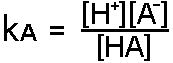In language, the equilibrium expression reads; "The dissociation constant of an
acid is equal to the concentration of hydrogen ions times the concentration of the
conjugate base of the acid divided by the concentration of un-ionized acid."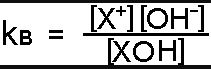Similarly, the equilibrium expression for a weak base reads: "The dissociation
constant of a base equals concentration of hydroxide ions times concentration of conjugate
acid divided by the concentration of un-ionized base."

The kA of an acid or the kB
of a base are properties of that acid or base at the given temperature. The temperature at
which these dissociation constants are listed is usually near room temperature.

The equilibrium expressions are for monoprotic acids or monobasic alkalis or the first
dissociation of a polyprotic acid or a polybasic alkali. Phosphoric acid (H3PO4) is a good example of a polyprotic acid. When completely ionized, a mol of phosphoric acid will give three hydrogen ions and a phosphate ion, but the hydrogen ions come off one at a
time at different pH’s and with different kA‘s.

 H3PO4 ===> (H2PO4)– + H+ first ionization kA = 6.92 E-3 (H2PO4)– ===> (HPO4)2– + H+ second ionization kA = 6.17 E-8 (HPO4)2– ===> (PO4)3– + H+ third ionization kA = 2.09 E-12

Any acid with more than one ionizable hydrogen or any base with more than one ionizable
hydroxide will usually separate stepwise as phosphoric acid.

## The pKa Box

The pKA of an acid is a very useful
number, as you will see in the math below. The pKA
is the negative log of the kA, the pKB
is the negative log of the kB, and the pKA
plus the pKB equal fourteen. The kA
box is the same as the pH box, but substitute kA
for [H+], pKA
for pH, kB for [OH],
and pKB for pOH.## pH of Strong Acids and Bases

Strong acids and bases have all of the dissolved material completely
ionized. The concentration of a monoprotic acid is equal to the concentration
of hydrogen ion. The concentration of a monobasic alkali is equal to the
concentration of  hydroxide ion. The actual concentration of hydrogen
ion (or hydroxide ion) from pure water is on the order of concentration of
E-7 Molar, so any concentration of a strong acid or base over E-5 Molar completely
swamps the comparatively tiny amount of ion from the ionization of water.

What is the pH of 0.0850 M HNO3? Nitric
acid is a monoprotic strong acid. [HNO3] = [H+] and pH = – log [H+],
so, pH = – log (0.085) = 1.07 Only one step on the pH box.

What is the pH of 0.00765 KOH? Potassium hydroxide is a monobasic strong base. [KOH] =
[OH] and  pOH = – log [OH] and pH = 14 – pOH Or you could go around the pH box the
other way. The pOH = 2.12 and pH = 11.88.

## Weak Acids

The following tables are published here for your convenience in working problems and
seeing examples of weak acids and bases. There is no need for you to memorize the names,
formulas, or numbers associated with these materials.

 ACID FORMULA kA pkA acetic acid H(C2H3O2) 1.74 E-5 4.76 ascorbic acid (1) H2(C6H6O6) 7.94 E-5 4.10 ascorbic acid (2) (HC6H6O6)– 1.62 E-12 11.79 boric acid (1) H3BO3 5.37 E-10 9.27 boric acid (2) (H2BO3)– 1.8 E-13 12.7 boric acid (3) (HBO3)= 1.6 E-14 13.8 butanoic acid H(C4H7O2) 1.48 E-5 4.83 carbonic acid (1) H2CO3 4.47 E-7 6.35 carbonic acid (2) (HCO3)– 4.68 E-11 10.33 chromic acid (1) H2CrO4 1.82 E-1 0.74 chromic acid (2) (HCrO4)– 3.24 E-7 6.49 citric acid (1) H3(C6H5O7) 7.24 E-4 3.14 citric acid (2) (H2C6H5O7)– 1.70 E-5 4.77 citric acid (3) (HC6H5O7)= 4.07 E-7 6.39 formic acid H(CHO2) 1.78 E-4 3.75 heptanoic acid H(C7H13O2) 1.29 E-5 4.89 hexanoic acid H(C6H11O2) 1.41 E-5 4.84 hydrocyanic acid HCN 6.17 E-10 9.21 hydrofluoric acid HF 6.31 E-4 3.20 lactic acid H(C3H5O3) 8.32 E-4 3.08 nitrous acid HNO2 5.62 E-4 3.25 octanoic acid H(C8H15O2) 1.29 E-4 4.89 oxalic acid (1) H2(C204) 5.89 E-2 1.23 oxalic acid (2) (HC2O4)– 6.46 E-5 4.19 pentanoic acid H(C5H9O2) 3.31 E-5 4.84 phosphoric acid (1) H3PO4 6.92 E-3 2.16 phosphoric acid (2) (H2PO4)– 6.17 E-8 7.21 phosphoric acid (3) (HPO4)= 2.09 E-12 12.32 propanoic acid H(C3H5O2) 1.38 E-5 4.86 sulfuric acid (2) (HSO4)– 1.05 E-2 1.98 sulfurous acid (1) H2SO3 1.41 E-2 1.85 sulfurous acid (2) (HSO3)– 6.31 E-8 7.20 uric acid H(C5H3N4O3) 1.29 E-4 3.89

The organic acids in the table above have been written as acid with
conjugate base as different from the standard notation. Notice that sulfuric
acid’s first ionization is a strong acid, so it is not on this table. Ascorbic acid
is vitamin C. Some of the acids in the table are not very soluble, such as
uric acid.

An organic acid usually has a – COOH group in it. The shape of that group is more like;

 O = C _ OH |

There is a double bond between the carbon and the single oxygen. There is
a single bond with an alcohol group (-OH). There is one more bond to the
central carbon that is usually attached to another carbon. The hydrogen of the
alcohol group is the one that has a tendency to ionize away from the group,
leaving a (-COO) ion at the end of the acid.

Notice the set of organic acids that begin with formic acid. Formic acid
has a hydrogen attached to the carbon in the organic acid group. Acetic
acid has a (-CH3) group attached
to the carbon in the organic acid group. The line of carbons becomes longer
for the acids that follow. There are no branched chains of carbon atoms or any
double or triple bonds between the carbons of the organic acids on this list.

The physiological organic acids (the organic acids that are found in living
things) all have even numbers of carbons. Hexanoic, octanoic, and decanoic (C-6,
C-8, and C-10 acids) are named for goats, which will give you an idea of the
smell of those compounds.

The name most used for the organic acid is in bold print in this table.

 SYSTEM NAME NUMBER OF CARBONS COMMON NAME methanoic acid 1 carbon formic acid ethanoic acid 2 carbons acetic acid propanoic acid 3 carbons propionic acid butanoic acid 4 carbons butyric acid pentanoic acid 5 carbons valeric acid hexanoic acid 6 carbons caproic acid heptanoic acid 7 carbons enanthic acid octanoic acid 8 carbons caprylic acid nonanoic acid 9 carbons pelargonic acid decanoic acid 10 carbons capric acid THE  FOLLOWING  ARE  ‘FATTY  ACIDS’ dodecanoic acid 12 carbons lauric acid tetradecanoic acid 14 carbons myristic acid hexadecanoic acid 16 carbons palmitic acid octadecanoic acid 18 carbons stearic acid eicosanoic acid 20 carbons arachidic tetracosanoic acid 24 carbons lignoceric

Below are some of the molecular formulas of some of the organic acids in
the stick and symbol form. The ionizable hydrogen is in blue.Organic acids with straight carbon even – number chains of twelve to twenty –
four are called fatty acids. You can see that the
pKA‘s are very close for all of the similar organic acids.
Formic and acetic acids are water soluble. As the carbon chain increases in
length, the molecule becomes less and less water soluble. Beginning with
propanoic acid, even as the number of carbons increase, the
pKA‘s do not change much.

## Weak Bases

Many materials are weak bases due to the presence of an amino group
-(NH2) attached to an organic compound. Nitrogen
has five electrons in the outside shell. The three solo electrons can participate
in (covalent) bonds to the nitrogen. The unshared pair of electrons can be donated
by the nitrogen atom to constitute a complete covalent bond with a material
that lacks a pair of electrons. The Lewis definition
most clearly shows that the donor of the pair of electrons (the N atom) is a
base and anything that attaches to the nitrogen by accepting a position of
covalent attachment to the nitrogen is an acid.

 BASE FORMULA kB pkB alanine C3H5O2NH2 7.41 E-5 4.13 ammonia (water) NH3 (NH4OH) 1.78 E-5 4.75 dimethylamine (CH3)2NH 4.79 E-4 3.32 ethylamine C2H5NH2 5.01 E-4 3.30 glycine C2H3O2NH2 6.03 E-5 4.22 hydrazine N2H4 1.26 E-6 5.90 methylamine CH3NH2 4.27 E-4 3.37 trimethylamine (CH3)3N 6.31 E-5 4.20

Alanine and glycine are amino acids, two of the twenty- or- so types of
building blocks of protein. Each amino acid has both an amino (base) end and
an acid end. Only the amino end (the base side) numbers are
listed.

## The 5% Rule

The measurement and calculation of pH is not as accurate as some other of
the chemical measures due to differences in temperature, other ions present,
purity of solutes, concentration changes due to evaporation, etc. Measurement
of pH in medicine, for instance must be at 37 degrees Celsius. There may be
machines that claim to measure pH to hundredths or thousandths of a pH unit,
but the standard that calibrates the machine may be off a little. A change of
five percent in the hydrogen ion concentration will not change the pH more than
a few hundredths of a pH unit. You try it on the calculator. Enter a number,
say 0.001 and punch log. Now punch a number 95% or 105% of the first
number (in this case, 0.00105 or 0.00095) and punch log. How different
is the pH? Our answers in pH are going to be to the nearest tenth of a pH unit,
so the hydrogen ion concentration needs to be only within 5% of an accurate
number. This idea is the ‘five percent rule.’

You may remember we mentioned that the concentration of a strong acid
is equal to the hydrogen ion concentration. That is not exactly so.
In any water solution of an acid there is another source of hydrogen ions, the
water. Water alone has a hydrogen ion concentration of E-7
Molar. Let’s say you have a E-5 M HCl solution. The hydrogen ion concentration from the acid is E-5, so the contribution of water ionization is only a hundredth of the amount of hydrogen ion from the acid. You could approximate the real hydrogen ion concentration
better by finding the hydroxide ion concentration of a solution with E-5
hydrogen ion concentration and then finding the added hydrogen ion concentration
due to the dissociation of water from that. The total hydrogen ion concentration
will be even further from having any significant contribution of hydrogen ion from
the ionization of water. Does the ionization of water have an significance in
this case? Of course not. There is much less than five percent difference
between the two numbers. Here is an obvious situation where you
can use the simpler approximation of the hydrogen ion concentration to find the
pH. There are some times when the simpler approximation is not accurate enough.
There are some times when you may be in doubt and would need to work it BOTH
ways to show whether you can use a simplified method.

Another way to state the five percent rule is: If you
have a simplified way of solving for the hydrogen ion concentration that would
give you an answer you can show would be within 5% of the more accurate way of
calculating it, you can use the simpler method.

## pH of Weak Acids and Bases

Weak acids and bases do not ionize very much, so the [H+]
or [(OH)] must be calculated by the equilibrium expression.

This would be easy to know exactly, except that the [HA] is not always equal
to the concentration of solute that actually went into the solution. The more
accurate way to express the equilibrium expression would be to include in the
denominator the idea that the [HA] is really the concentration of solute
originally put into solution minus the amount of that solute that ionized. We
could represent the amount that ionized by either the [A]
or the [H+],
so the denominator should be either [HA] – [A] or [HA] –
[H+]. Let’s use the
second option for alternative denominator because we want to solve for the hydrogen ion concentration. By
the 5% rule, [HA] = [HA] – [H+]
only if the hydrogen ion concentration is less than five percent of the total
solute concentration. This is the case for most weak acids. The only exceptions
are when the strongest of the weak acids are in the most dilute solutions.

If you have a stronger weak acid (one with a high kA),
you should check to see if the [H+] is near 5% of the
total solute concentration. If it is, you will have to use the alternative
denominator in the equation and solve it for [H+] by quadratic
equation.Heaven forefend the need for using a quadratic equation. (I would rather kiss
a BIG alligator.) It’s a lot easier if the [H+]
is less than five percent of the [HA]. The calculation of pH of
VERY weak acids like boric acid always requires the use of a quadratic equation, but let’s avoid that in the basic course.

If there is only water and a weak acid in the solution, each mol of the
acid dissociates into only one mol of hydrogen ions and only one mol of
conjugate base ions. [H+]
= [A] ! So;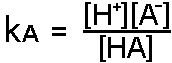and solving for [H+]2,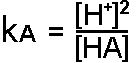then taking the square root of both sides, you have [H+].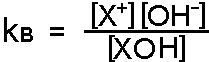Once you have the hydrogen ion concentration, you can go anywhere on the pH box.

The same idea goes for a weak base as the only material in water solution. Start with
the equilibrium expression and the conjugate acid ion being equal to the hydroxideand solve for [OH] to get;And it’s back to the pH box for anything else you need.

## Buffers and pH of Buffers

A buffer is a solution that resists changes in pH. A buffer is made with a
weak acid and a soluble salt containing the conjugate base of the weak acid
or a weak base and a soluble salt containing the conjugate acid of the
weak base. Some examples of buffer material pairs are:

acetic acid and sodium acetate, H(C2H3O2) and Na(C2H3O2)

hydrofluoric acid and potassium fluoride, HF and KF

carbonic acid and sodium bicarbonate, H2CO3 and NaHCO3

ammonium hydroxide and ammonium nitrate, NH4OH and NH4NO3

nitrous acid and lithium nitrite, HNO2 and LiNO2

Consider the above pairs. There are four acid buffers and one base (alkaline) buffer. The acids all show a hydrogen that is singly ionizable and its conjugate base, such as HF reversibly ionizes to H+ and F. The HF is the weak acid and the fluoride ion is the conjugate base. We sometimes say that the KF is the conjugate base, but the identity of the anion in the conjugate DOES NOT MATTER except as we weigh the compound to include in the buffer. In the base buffer, the anion, in this case ammonium, is the conjugate.

To explain how a buffer works, let’s use the example of an acid buffer. The weak acid of a buffer in solution alone in the water would be more acid than the buffer. The conjugate base in the buffer alone in water would be more alkaline, but the two can exist TOGETHER in the solution without neutralizing each other. THE BUFFER PAIR CANNOT REACT WITH EACH OTHER BECAUSE THEY HAVE A COMMON ION, THE CONJUGATE. When more (stronger) base is added to the buffer solution, some of the acid gets neutralized, making more of the conjugate base. When (stronger) acid is added to the buffer, it neutralizes the conjugate base, making more of the weak acid. As long as there is both some weak acid and conjugate base, the buffer still works and the pH is not very far from the pKA of the acid.

A buffer is most effective in solutions of pH at or close to the pKA of
the weak acid. (Or solutions of pOH at or close to the pKB of the weak base.)
The most buffering capacity is available when the concentration of weak acid
or base is close to the concentration of the conjugate ion and when the
concentration of both is greatest. A one molar buffer, when both parts of the buffer are one molar, has double the buffering capacity of a half molar buffer.

Of what use is a buffer for biological systems? Living things require a very close homeostasis, a regular temperature, osmotic pressure, pH, etc. in order to stay alive. If a person has too much acid, the condition is called acidosis. If too much base, alkalosis. Either condition is life – threatening. There are several buffer systems in the body, a phosphate system, a carbonate system, and a protein system. The major inorganic systems, phosphate and carbonate, depend on the phosphate – biphosphate or carbonate – bicarbonate concentrations. (The concentration of carbon dioxide in the blood is a part of the carbonate system.) The protein system depends on the weak acid and base groups on the outside of proteins, mainly globular proteins in the blood.

Thinking backwards, if you need a buffer at a particular pH lower than
seven, chose the weak acid that has a pKA
close to that pH and a conjugate base to go with it.

We can start again from the equilibrium expression of the ionization of a
weak acid or a weak base;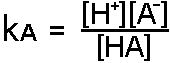In buffer solutions the concentration of hydrogen ion ([H+])is not equal
to the concentration of conjugate ion ([A]). The concentration of conjugate ion from
the dissociation of the acid is pretty much negligible, so we can calculate as if all the
conjugate ion comes from the salt. If the soluble salt has only one mol of
conjugate ion per mol of salt, the concentration of conjugate ion is the same
as the concentration of salt.

The entrance to the pH box again is the hydrogen ion concentration. Solve
for the hydrogen ion concentration if you need it, the pH of the solution, the
pOH, or the hydroxide ion concentration.The Henderson-Hasselbalch (H-H) equation can be somewhat confusing, but it is
nothing more than the negative log of the equation above, the
equilibrium expression solved for the hydrogen ion concentration. If you know
the equilibrium equation, know how to solve the equilibrium equation for the
hydrogen ion concentration, and know how to convert the hydrogen ion
concentration to the pH, you have it licked. If you really need the H-H
equation for a test, it is best to make sure you are getting it right by
deriving it from the equilibrium expression.

-log[H+] = pH

-logkA = pKA

-log(1/[A]) = +log[A]

-log([HA]) = -log[HA]

Component-by-component, take the negative log of the equilibrium expression
and change the multiplication to addition. (They are logs now.) You get:

pH = pKA +
log[A] – log[HA]
, which is the same as;

pH = pKA – log([HA]/[A]
)
, the usual way you see the H-H equation.

There are four perfectly correct ways to write the H-H equation. They are: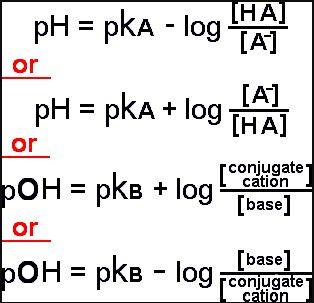An equimolar buffer is one in which the concentration of
the weak acid (or base) is the same as the concentration of the conjugate ion.
This may not seem particularly significant to you, but there are several
important ideas that can be easily seen from it. Start with the ionization
equilibrium expression and cancel the [HA] with the [A]. This shows
that in an equimolar buffer kA
= [H+].This shows how a buffer works. As acid or base is added to a buffer solution,
the buffer equilibrium will trade off [HA] with [A] to come to a
new equilibrium. When that happens, there will be a much smaller change in the
hydrogen ion concentration than if the acid or base were added to an unbuffered
solution.

Now let’s do a similar trick with the H-H equation. If [HA] = [A],
the term [HA]/[A] = 1 and log 1 = 0, so pH =
pKA.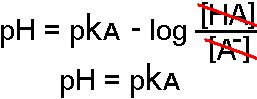This indicates that in an equimolar buffer the pH = pKA
and that the RATIO of the [HA] to the [A] will determine the pH of
the solution. The further the ratio gets from one to one, the further the pH
gets from the pKA. The buffer has
its greatest buffering power at the pKA
of the weak acid (or base). The higher the concentration of both the weak acid
and its conjugate ion, the more buffering power is available.## Titration

The word "titration" rhymes with "tight nation." The medical use of this word is a little different, but in chemistry titration refers
to a commonly used method of finding the concentration of an unknown
liquid, by comparing it with a known liquid (or known mass of solid in solution). An acid-base titration is good to
consider when learning the method, but there are more uses for the technique.
The measure of oxalate ion using potassium permanganate in a warm acid environment
is a good example of a redox titration. The Mohr titration is a determination
of chloride concentration using known silver nitrate solution and sodium dichromate
an indicator.

A measured amount of the unknown material in a flask with indicator is usually
combined with the known material from a buret (rhymes with "sure bet"). The buret
is marked with the volume of liquid by a scale with zero on top and (usually)
fifty milliliters on the bottom. The buret has some type of valve at the bottom
that can dispense the contained liquid.

It is not necessary to start the titration with the known liquid level in the
buret at the zero mark, but the level must be within the portion of the buret that
is marked. The buret on the left shows about 1.7 ml of the yellow liquid in
it because the bottom of the meniscus is between the 1 and 2 mL markings and closer to the
2 mL mark. Most laboratory burets can be read to an accuracy of one hundredth of a
milliliter. (The drawing on the left is a bit crude. Most burets show the ten
divisions of a milliliter and you can interpolate between the marks.) One reads
the buret by getting at eye level to the bottom of the meniscus (curve in the
liquid) and comparing the bottom of the meniscus to the marks on the glass.
A reading of the buret is taken before and at the end of the titration. The
amount of know – concentration liquid used is the difference of the beginning and

The endpoint of the titration is usually shown by some type of indicator.
A pH indicator is a material, usually an organic dye, that is one color above
a characteristic pH and another color below that pH. There are many materials that can
serve as pH indicators, each with its own ph range at which it changes color. Some have
more than one color change at distinct pH’s. Litmus and phenolphthalein are
common pH indicators. Litmus is red in acid (below pH 4.7) and blue in base (above pH 8.1).
Phenolphthalein (The second ‘ph’ is silent and the ‘a’ and both ‘e’s are long, if that is any help.)
is clear in acid (below pH 8.4) and pink- purple in base (above pH 9.9). These ranges may
seem large, but near the equivalence point, the point at which the materials
are equal, there is a large change in pH. The equivalence point may not occur at pH 7,
neutral pH, so the appropriate pH indicator must be chosen for the type of acid and
base being titrated.

The volume of the material of unknown concentration is known by how much is put into the
reaction vessel. The concentration of the standard is known, and its volume is known
from the measurement of liquid used in the titration.

If you have a monobasic base and a monoprotic acid, the titration formula can be simplified to:

if CA =
the concentration of the acid and CB =
the concentration of the base and VA =
the volume of acid solution and VB =
the volume of base, then,Some textbooks use the symbol “M” for concentration in units of Molar, so they would show the formula as MA VA
MB VB.

## Salts

A salt is the combination of an anion (- ion) and a cation (a
+ ion). Another way to think of a salt is the combination of the anion of a
certain acid combined with the cation of a certain base. The neutralization
of potassium hydroxide with hydrochloric acid produces water and the salt,
potassium chloride. In a solid salt, the ions are held together by the difference in charge. Solid salts
usually make crystals, sometimes including specific molar amounts of water,
called water of hydration into the crystal. If a salt dissolves in
water solution, it usually dissociates (comes apart) into the anions and
cations that make up the salt.

Salts dissolved in water may not be at neutral pH. Table salt, NaCl, has a
neutral pH in water, but baking soda, NaHCO3 is very
alkaline when dissolved in water. Take a teaspoonful of baking soda on your hand,
wet it, and completely wash your hands with it. (Baking soda is a really fine
material for cleaning hands!) There is the hint of the slipperiness of bases on

How can you predict the pH of a salt dissolved in water? The actual pH will
depend on the type of anion and cation, the solubility of the salt, the temperature
of the solution, and concentration of the salt if less than saturated.
Here are the general rules:

Salts made of the anion of a strong acid and the cation of a strong base
will be neutral salts, that is, the water solution with this salt will have
a pH of seven. (Example – sodium chloride)

Salts made of the anion of a strong acid and the cation of a weak
base will be acid salts, that is, the water solution with this salt will have
a pH of less than seven. (Example – ammonium chloride)

Salts made of the anion of a weak acid and a strong base will be
an alkali salt. The pH of the solution will be over seven. (Example – sodium bicarbonate)

It can be a bit more difficult to tell the pH of a salt solution if the
salt is made of the anion of a weak acid and the cation of a weak base. Usually,
the main determining factor is whether the weak acid is weaker than the weak base,
but that is not always the case. For the purpose of the problems in the review
section, you may say that the pH of a ‘weak-weak’ salt is indeterminate.

## Titration and pH Problems

THE FOLLOWING SALTS IN WATER SOLUTION WILL HAVE A pH OF 7, < 7(less than 7), > 7(more
than 7), OR INDETERMINATE (I). FOR EACH PREDICT THE pH OF THE SOLUTION.

 1. Na2CO3 2. FeCl3 3. KNO3 4. NH4C2H3O2 5. ZnSO4 6. Ba(NO3)2 7. RbF 8. CaBr2

FIND THE pH OF THE FOLLOWING SOLUTIONS. Find kA’s, pKA’s,
kB’s, and pKB’s on the tables
above.

 9. 0.0115 M HCl 10. 0.0815 M NaOH 11. 0.00372 M Ba(OH)2 12. 0.12 M HC2H3O2 13. 1.35 E-5 H3BO3 14. 0.255 M NH4OH 15. 0.578 M H3PO4 16. 0.16 M HCl and 0.072 M phosphoric acid. 17. 1.25 M acetic acid and 0.75 M potassium acetate. 18. 0.788 M lactic acid and 1.27 M calcium lactate. 19. 0.590 M ammonium hydroxide and 1.57 M ammonium chloride. 20. Explain how to make 5 L of 0.15 M acetic acid-sodium acetate buffer at pH 5.00 if you have 1.00 Molar acetic acid and crystalline sodium acetate.

FOLLOWING ARE TITRATION PROBLEMS. ASSUME THAT THE pH INDICATOR WILL BE THE RIGHT ONE TO
BALANCE THE AMOUNT OF ACID AND BASE. SOME OF THE TITRATIONS ARE NOT ACID –  BASE
TITRATIONS. AGAIN ASSUME THERE IS AN INDICATOR THAT WILL TELL WHEN MOLAR AMOUNTS ARE
MATCHED.

21. 23.45 mL of 0.275 M sodium hydroxide was used to titrate against mL of acetic acid.
What was the concentration in M of acetic acid?

22. 17.05 mL of 0.247 M barium hydroxide was used to titrate against 10 mL of nitric
acid. What was the concentration in M of nitric acid?

23. 35.79 mL of 0.275 M sodium hydroxide was used to titrate against 15 mL of sulfuric
acid. What was the concentration in M of sulfuric acid?

24. 24.92 mL of 0.00199 M silver nitrate was used to titrate against 5 mL of sodium
chloride solution. What was the concentration of NaCl?